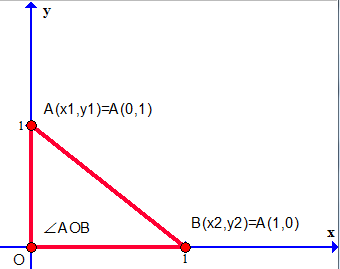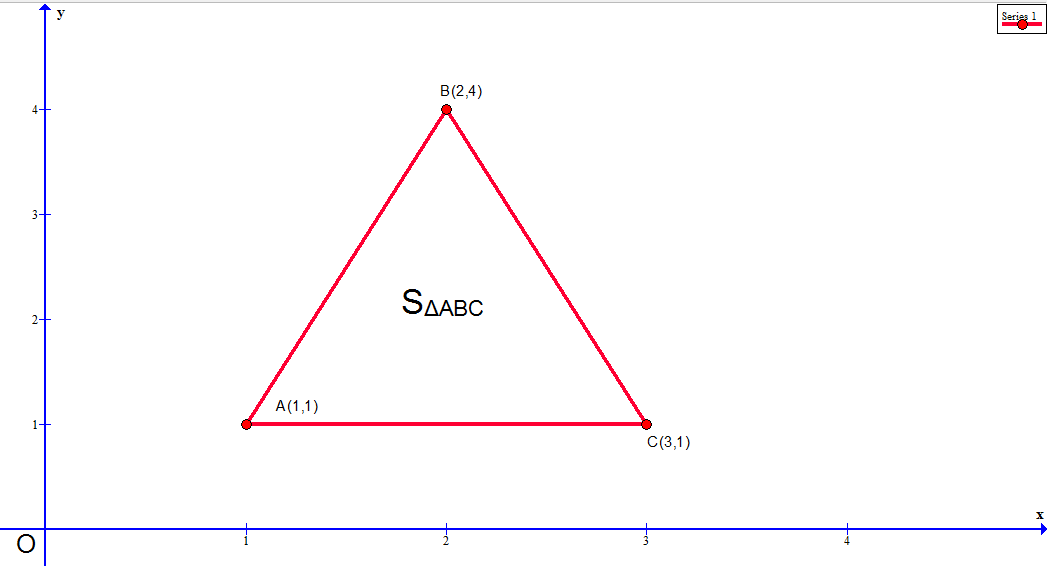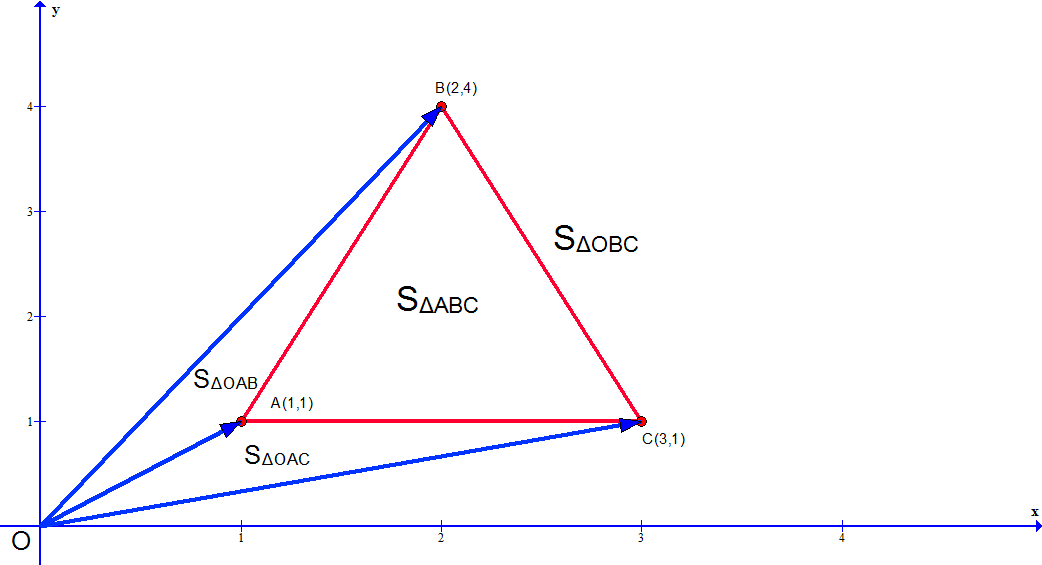# 由顶点坐标计算任意多边形面积SABC =0.5× |OA|×|OB|×sin（∠AOB）
=0.5×|OA×OB|
=0.5×|(x1,y1)×(x2,y2)|
=0.5×[(x1y2)-(y1x2)]=SABC = SOBC + (-SOAC) + (-SOAB)

SABC=0.5×|(x1y2-x2y1)+(x2y3-y2x3)+(x3y1-y3x1)|

S = 0.5×|(x1y2-x2y1)+(x2y3-y2x3)+(x3y4-y3x4) + ...... + (xny1-ynx1)|

posted @ 2019-02-17 11:20  Velscode  阅读(1887)  评论(0编辑  收藏